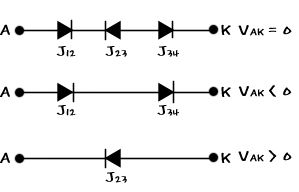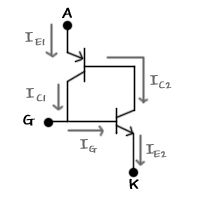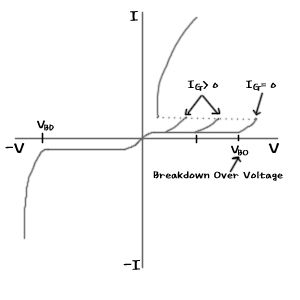top of page
Search

# SCR,TRIAC,DIAC

After PN junction diode -> BJT -> JFET -> MOSFET, I will try to summarize SCR (Silicon Controlled Rectifier).

Now, I think this is the last time for the device related to the PN junction.

If the PN junction diode is 1 junction, then BJT is 2 junction... JFET or MOSFET is also 2 junction...

From a point of view, it is not possible to say that MOSFET is also two junction...

So, this time, let's summarize the three-junction element SCR, in other words a Thyristor.

Actually, it can be called a special device.

It is a device frequently used for power circuit control or AC control.

As mentioned above, since it is a three-junction, the structure is a P-N-P-N type three-junction four-layer structure.

In addition to the Thyristor, there are also others called DIAC and TRIAC.

Let’s take a look one by one~

First, the circuit diagram is as follows.Looking at the picture, there are some kind of diode-like pictures drawn here and there, and there's also one more G.

A and K refer to the Anode and Cathod that we have seen a lot. G is the Gate that we only saw in FET.

uh? But for some reason, there is a Gate, so I feel right...

That's right~ Right~ The role of Gate is to control the flow of current.

But~ If it performs the same function, it is no different from BJT and FET~!

Then, let's talk about what's different by looking at the Thyristor structure below.As mentioned above, the biggest feature of Thyristor is that it has a 3-junction structure with PNPN.

In addition, each part has 3 terminals as the anode, cathode and gate part as shown in the picture above.

To put it simply, if you divide each PNPN junction structure according to the voltage applied between the anode and the cathode,

You can think of it as a structure in which diodes are connected as shown in the picture below.

In other words, if you do not apply any voltage with Vak=0, you can think of it as a diode structure of J12-J23-J34.

Also, if Vak <0, J12 and J34 are in reverse bias, and only J23 is forward biased.

You can think of it like a second diode-coupled shape...

If Vak> 0, J12 and J34 are forward biased, so I think that they are short, and J23 is reverse bias, so it remains alone.

Even if you look at this explanation, even if it is a PNPN structure, if you think about it separately, it doesn't feel difficult and the interpretation seems to be perfect.

If so, let's look at the structure in which Vak> 0 and Vg> 0 are connected above.

For actual analysis, it can be divided into PNP / NPN structures as shown on the right. In other words, PNP TR and NPN TR are

You can think of it as a connected structure.If you look at the process of going on-state between A-K...

1) If Ig = 0 at the beginning, both PNP / NPN TR are kept off and current does not flow between A and K.

2) Here, if Ig> 0 and current flows through the gate, current flows into the base of NPN TR and NPN TR is On, allowing Ic2 and Ie2 to flow.

3) Because Ic2 can flow, current can flow to the base of PNP, so PNP is also On, and as a result, Ic1 can flow.

4) This Ic1 is now connected with Ig, so even if the gate current is set to 0, there is a current of Ic1, so the current can continue to flow between A and K as a whole.

Because of this structure, once the SCR is On-state, it can keep the On state regardless of the Gate signal.

So, to return to the Off state, open the Anode section so that no more current can flow.

The voltage between A-K must be reverse biased (reverse voltage state) to make it off-state.The above graph is the I-V characteristic curve graph of SCR..

At a glance, you can see that there is no difference from the characteristics of a general diode in the reverse bias state.

Conversely, even in the forward bias state, if Ig = 0, we can see that it is similar to the reverse bias.

In other words, when Ig = 0, the time when the J23 diode goes into the avalanche state in the figure above is Vbo, breakdown over voltage, and the current suddenly flows between A-K.

Also, if Ig> 0, the value of Vbo decreases, so that current flows between A and K in the smaller Vbo.I think it is easy to see that the I-V characteristic curves of DIAC and TRIAC appear similar to the I-V characteristic of SCR above.

In the case of DIAC, the gate is removed from the SCR, so the current is increased by turning on-state at break over voltage and break down voltage respectively.

In TRIAC, SCRs are attached in parallel and gates are used in common, so it is easy to understand if you think that the forward bias characteristic curve of SCR is visible in both directions.The above picture shows the input of SCR and the signal of the gate, and the corresponding output signal. When AC signal is put into the input as above, if you give a light to the gate, the signal continues to flow even if the gate signal is cut off. When the polarity is reversed and the reverse bias is made, the SCR enters the off-state again and the signal is not visible at the output stage.

In other words, as shown in the picture above, only the signal that was cut in the + direction is visible.

If TRIAC instead of SCR is used here, and the gate signal puts a reverse pulse, the reverse biased input signal can be output at the output stage.

In this way, it is possible to control AC signals by using devices such as SCR or TRIAC. In other words, it is difficult to control the AC signal with a TR, but it is possible to control it with an element such as SCR.

In addition, if you use the above characteristics, you can control the speed of the AC motor (the same effect as controlling the speed of the motor with PWM) and the current of the device using AC.

For reference, there is a GTO (Gate Turn Off) type SCR. This SCR is a SCR that turns the SCR that keeps ON continuously once turned ON when the Gate signal applies a reverse bias signal to the OFF mode. Also know that there is

Ah.. And if you connect a device such as DIAC to the line of the power or signal side as follows...It's good to know that it can be used as a protection circuit because DIAC becomes conductive when a sudden high voltage such as a surge voltage is applied.